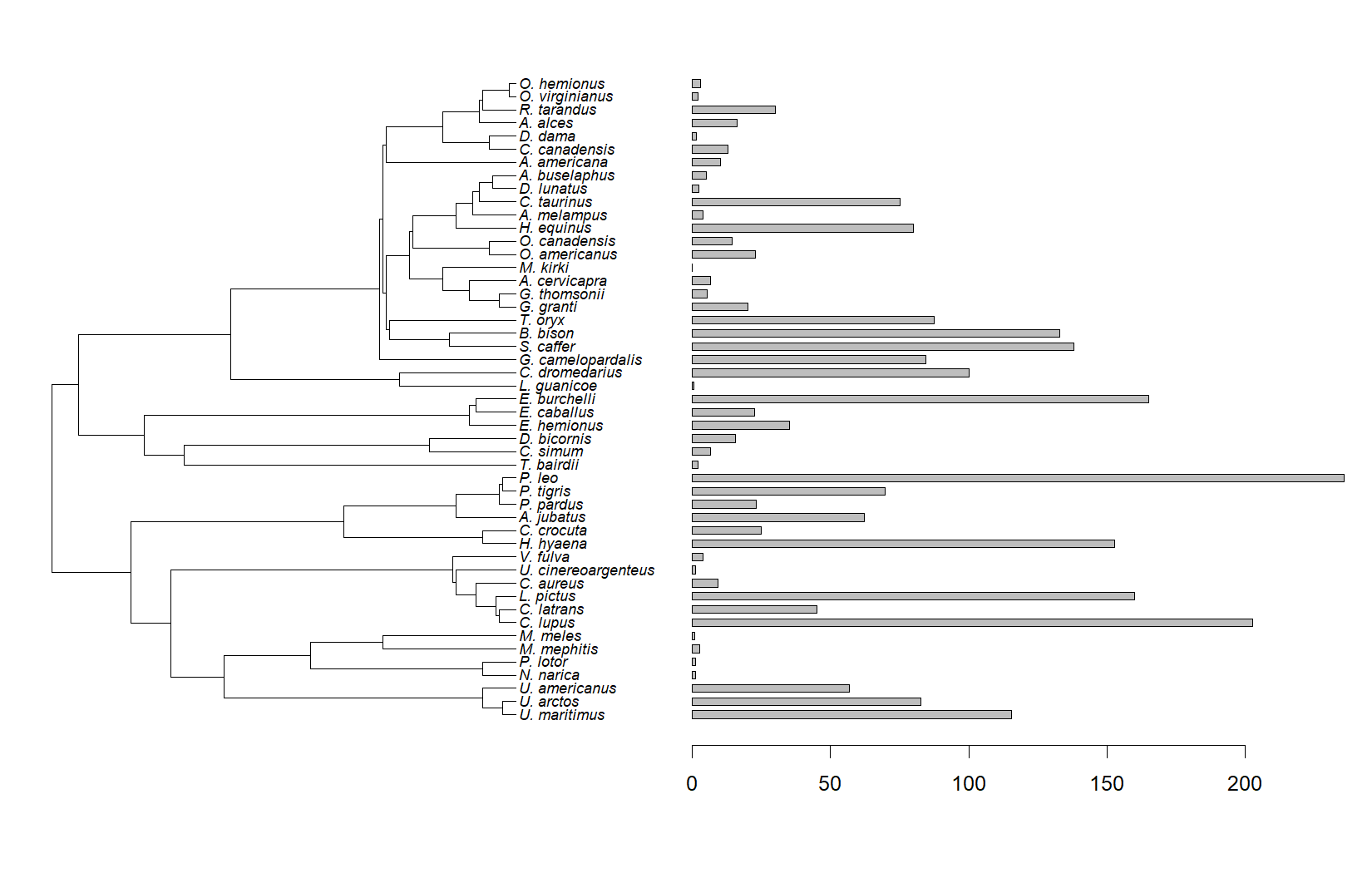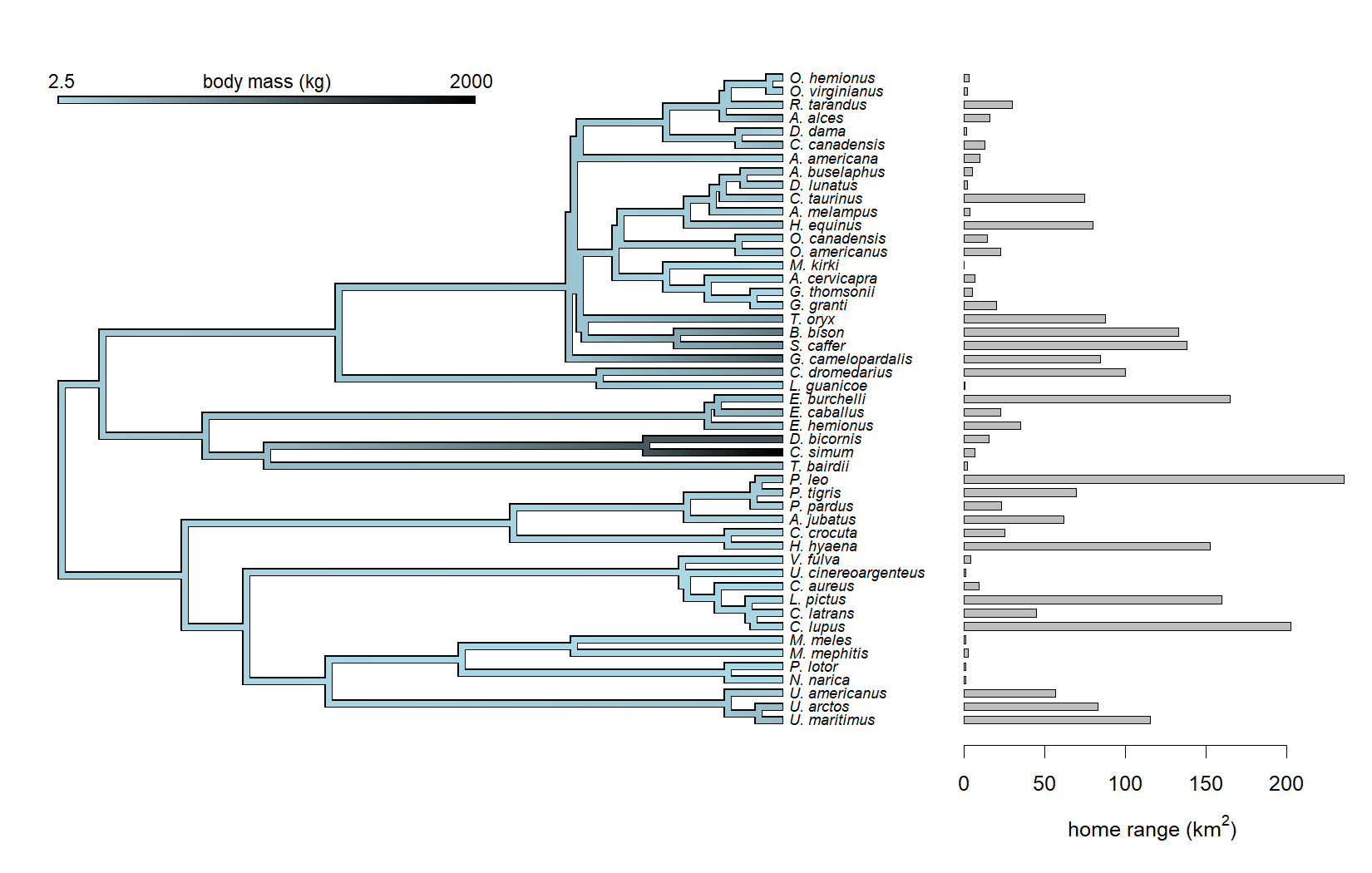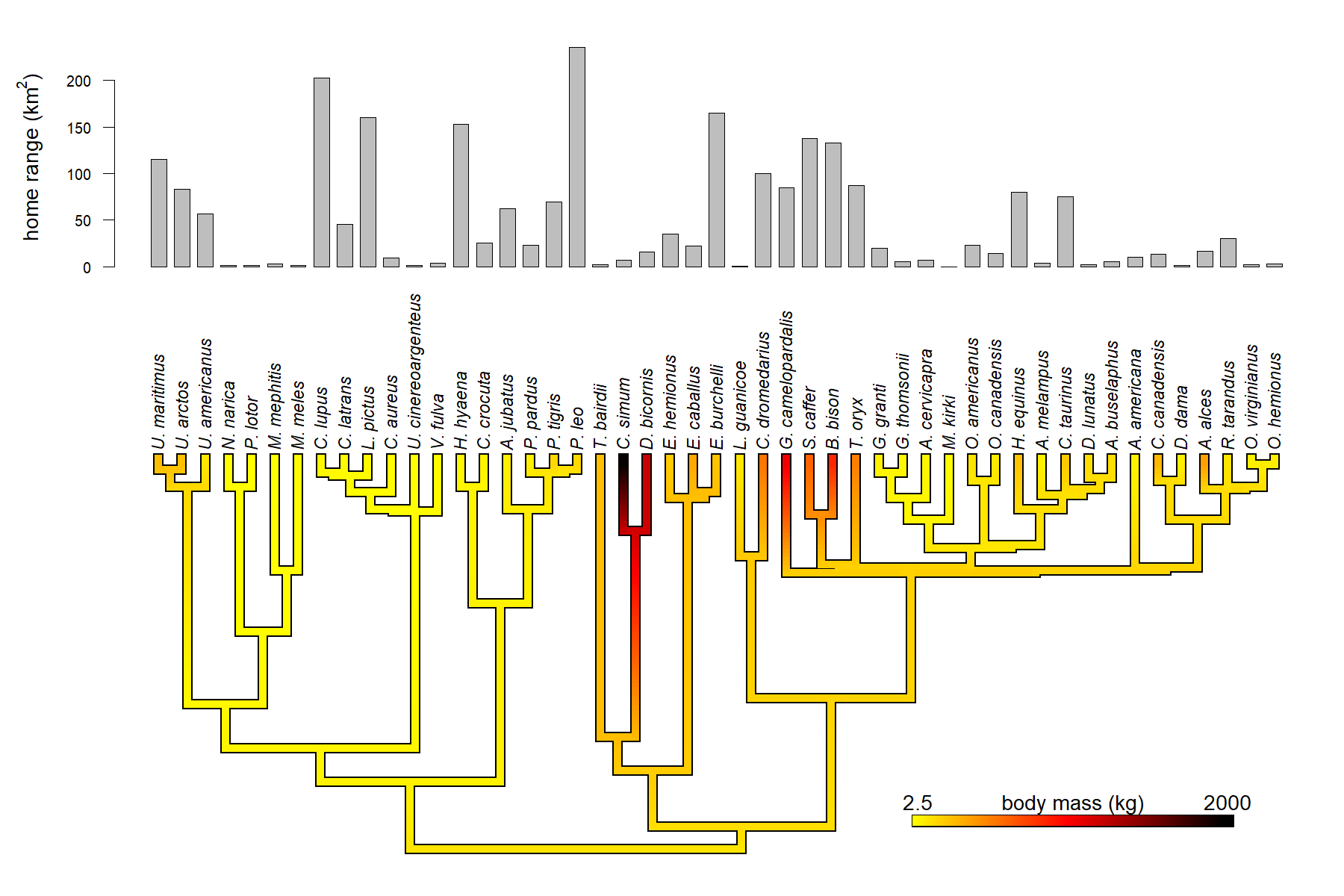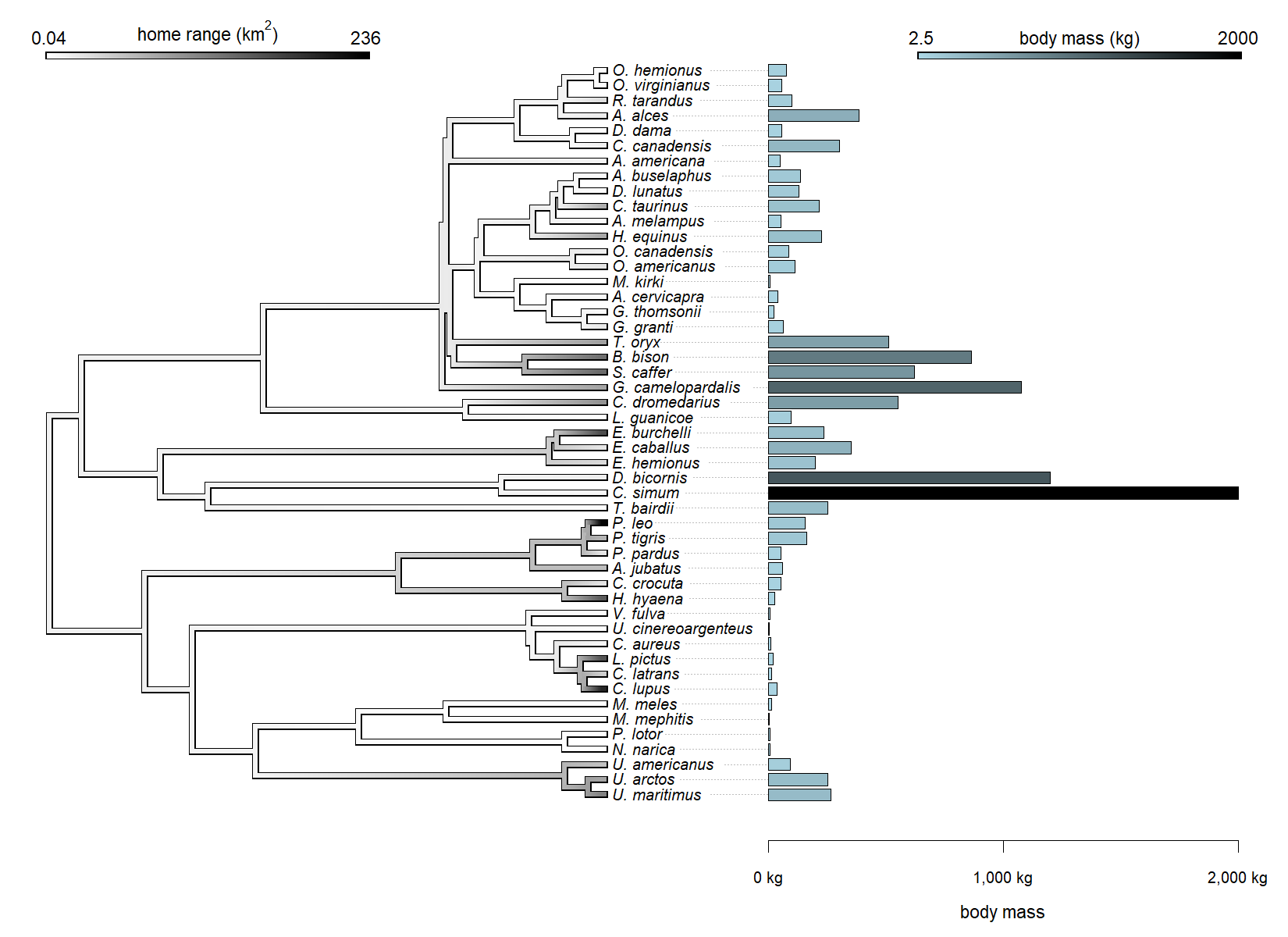## Friday, July 8, 2022

### Adding a custom barplot at the tips of a plotted tree in R

Last week, a phytools user sent me the following request:

“Do you know whether or not there is a function similar to `tiplabels` but that allows to add tip labels that represent a continuous character in a phylogeny that is already printed. I am looking for something like your `plotTree.wBars` but that allows me to add the bars in a tree that is already plotted.”

It turns out that they found an alternative solution, but I just wanted to give a quick demo because (of course) this is possible.

To see how, let's take the following data:

``````library(phytools)
data(mammal.tree)
mammal.tree
``````
``````##
## Phylogenetic tree with 49 tips and 48 internal nodes.
##
## Tip labels:
##   U._maritimus, U._arctos, U._americanus, N._narica, P._lotor, M._mephitis, ...
##
## Rooted; includes branch lengths.
``````
``````data(mammal.data)
``````
``````##               bodyMass homeRange
## U._maritimus     265.0    115.60
## U._arctos        251.3     82.80
## U._americanus     93.4     56.80
## N._narica          4.4      1.05
## P._lotor           7.0      1.14
## M._mephitis        2.5      2.50
``````

We can imagine that we'd like to make a `"contMap"` style visualization of our body mass data, but then superimpose at the tips of the tree a set of bars representing our home range sizes.

I choose a `"contMap"` visual just because it is not one that automatically permits a bar graph to be plotted at the tips.

There are many ways to do this, of course. Here I'll try to demonstrate a few.

To begin with, I'm going to pull out our two trait vectors from the data frame as follows.

``````## pull out body mass
bodyMass<-setNames(mammal.data\$bodyMass,
rownames(mammal.data))
## pull out range size
homeRange<-setNames(mammal.data\$homeRange,
rownames(mammal.data))
``````

For the first method, perhaps, let's use `layout` and split our plot.

In this case, I'll just plot my `"contMap"` graph in the left panel, and my bars on the right.

I have to use `plot.simmap` to graph my `"contMap"` plot so I can adjust the spacing of the tip labels to match my bar graph.

``````## do ancestral state estimation on a log-scale
fitted<-fastAnc(mammal.tree,log(bodyMass))
## compute a contMap object on original scale
cMap<-contMap(mammal.tree,bodyMass,method="user",
anc.states=exp(fitted),plot=FALSE)
## re-color contMap
cMap<-setMap(cMap,c("lightblue","black"))
obj<-plotTree.barplot(mammal.tree,homeRange,
args.plotTree=list(fsize=0.7))
````````````## split plotting area
layout(matrix(c(1,2),1,2),widths=c(0.7,0.3))
plot(cMap\$tree,cMap\$cols,fsize=0.7,
mar=c(5.1,1.1,2.1,0),tips=obj,
lwd=5,outline=TRUE,ylim=range(obj),
ftype="i")
lims=cMap\$lims,digits=2,prompt=FALSE,x=0,y=80,
subtitle="",lwd=5,fsize=0.9)
plotTree.barplot(mammal.tree,homeRange[mammal.tree\$tip.label],
args.plotTree=list(plot=FALSE),
args.barplot=list(xlab=
expression(paste("home range (km"^"2",")"))),
``````We can actually do this plotting our tree in an upward direction too. Just to keep things interesting, let's change the color palette of our `"contMap"` object.

``````## change color palette of contMap object
cMap.recolored<-setMap(cMap,c("yellow","red","black"))
## compute horizontal positions of bars
obj<-barplot(homeRange[mammal.tree\$tip.label],space=0.5,
plot=FALSE)
## split plotting arear
layout(matrix(c(2,1),2,1),heights=c(0.3,0.7))
## plot tree
plot(cMap\$tree,cMap.recolored\$cols,fsize=0.9,
mar=c(1.1,5.1,0,1.1),tips=obj,lwd=7,
outline=TRUE,ftype="i",
direction="upwards",xlim=range(obj),
offset=1)
lims=cMap\$lims,digits=2,prompt=FALSE,x=50,y=5,
subtitle="",lwd=9,fsize=1.1)
par(mar=c(0,5.1,2.1,1.1))
barplot(homeRange[mammal.tree\$tip.label],names.arg="",
xlim=range(obj),
ylab=expression(paste("home range (km"^"2",")")),
space=0.5,las=2,cex.axis=0.8,cex.lab=1.1)
``````Now, last of all, let's forgo use of `barplot` altogether, and just manually add some rectangles (proportional in length to the trait value) on to the tips of the tree.

This time, I'll create a `"contMap"` plot of range size, and graph body mass onto the tips of the tree.

``````## compute ancestral states of range size on a log scale
fitted<-fastAnc(mammal.tree,log(homeRange))
## compute a contMap object on original scale
cMap.homeRange<-contMap(mammal.tree,homeRange,method="user",
anc.states=exp(fitted),plot=FALSE,res=1000)
## re-color contMap
cMap.homeRange<-setMap(cMap.homeRange,c("white","black"))
## plot our contMap
plot(cMap.homeRange,fsize=0.8,legend=FALSE,xlim=c(0,150),
ylim=c(0,1.05*Ntip(mammal.tree)),mar=c(4.1,0.1,0.1,0.1))
title=expression(paste("home range (km"^"2",")")),
lims=cMap.homeRange\$lims,digits=2,prompt=FALSE,x=0,
y=1.02*Ntip(mammal.tree),subtitle="",lwd=5,
fsize=0.9)
## for fun I'll use the colors from my first contMap plot
xx<-max(nodeHeights(mammal.tree))+
0.9*max(strwidth(mammal.tree\$tip.label))
scale<-(150-xx)/max(bodyMass)
scale
h<-sapply(1:Ntip(mammal.tree),nodeheight,
for(i in 1:Ntip(mammal.tree)){
lines(c(h[i],xx),rep(i,2),lty="dotted",col="grey")
x<-xx+c(0,scaledBodyMass[i],scaledBodyMass[i],0)
y<-i+c(-0.4,-0.4,0.4,0.4)
COL<-cMap\$cols[round((scaledBodyMass[i]-min(scaledBodyMass))/
diff(range(scaledBodyMass))*1000)]
polygon(x,y,col=COL)
}
axis(1,at=xx+c(0,1000,2000)*scale,
labels=c("0 kg","1,000 kg","2,000 kg"),cex.axis=0.8)
mtext("body mass",1,line=2.5,at=mean(c(xx,150)),cex=0.9)
title="body mass (kg)",
lims=cMap\$lims,digits=2,prompt=FALSE,
x=150-40,
y=1.02*Ntip(mammal.tree),subtitle="",lwd=5,
fsize=0.9)
``````That's cool, right?